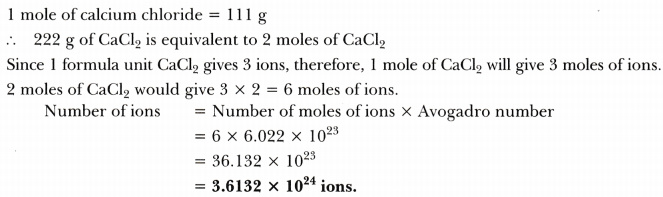# Calcium chloride when dissolved in water dissociates into its ions according to the following equation. CaCl2 (aq)----->Ca2+ (aq) + 2CI- (aq)

Calcium chloride when dissolved in water dissociates into its ions according to the following equation.
CaCl2 (aq)----->Ca2+ (aq) + 2CI- (aq)
Calculate the number of ions obtained from CaCl2 when 222 g of it is dissolved in water.